x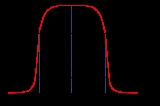BandwidthEncyclopedia
Bandwidth is the difference between the upper and lower frequencies in a contiguous set of frequencies. It is typically measured in hertz
Hertz
The hertz is the SI unit of frequency defined as the number of cycles per second of a periodic phenomenon. One of its most common uses is the description of the sine wave, particularly those used in radio and audio applications....

, and may sometimes refer to passband bandwidth, sometimes to baseband bandwidth, depending on context. Passband bandwidth is the difference between the upper and lower cutoff frequencies of, for example, an electronic filter
Electronic filter
Electronic filters are electronic circuits which perform signal processing functions, specifically to remove unwanted frequency components from the signal, to enhance wanted ones, or both...

, a communication channel, or a signal spectrum. In case of a low-pass filter
Low-pass filter
A low-pass filter is an electronic filter that passes low-frequency signals but attenuates signals with frequencies higher than the cutoff frequency. The actual amount of attenuation for each frequency varies from filter to filter. It is sometimes called a high-cut filter, or treble cut filter...

or baseband
Baseband
In telecommunications and signal processing, baseband is an adjective that describes signals and systems whose range of frequencies is measured from close to 0 hertz to a cut-off frequency, a maximum bandwidth or highest signal frequency; it is sometimes used as a noun for a band of frequencies...

signal, the bandwidth is equal to its upper cutoff frequency. The term baseband bandwidth always refers to the upper cutoff frequency, regardless of whether the filter is bandpass or low-pass.

Bandwidth in hertz is a central concept in many fields, including electronics
Electronics
Electronics is the branch of science, engineering and technology that deals with electrical circuits involving active electrical components such as vacuum tubes, transistors, diodes and integrated circuits, and associated passive interconnection technologies...

, information theory
Information theory
Information theory is a branch of applied mathematics and electrical engineering involving the quantification of information. Information theory was developed by Claude E. Shannon to find fundamental limits on signal processing operations such as compressing data and on reliably storing and...

Radio is the transmission of signals through free space by modulation of electromagnetic waves with frequencies below those of visible light. Electromagnetic radiation travels by means of oscillating electromagnetic fields that pass through the air and the vacuum of space...

communication
Communication
Communication is the activity of conveying meaningful information. Communication requires a sender, a message, and an intended recipient, although the receiver need not be present or aware of the sender's intent to communicate at the time of communication; thus communication can occur across vast...

s, signal processing
Signal processing
Signal processing is an area of systems engineering, electrical engineering and applied mathematics that deals with operations on or analysis of signals, in either discrete or continuous time...

, and spectroscopy
Spectroscopy
Spectroscopy is the study of the interaction between matter and radiated energy. Historically, spectroscopy originated through the study of visible light dispersed according to its wavelength, e.g., by a prism. Later the concept was expanded greatly to comprise any interaction with radiative...

.
A key characteristic of bandwidth is that a band of a given width can carry the same amount of information, regardless of where that band is located in the frequency spectrum
Frequency spectrum
The frequency spectrum of a time-domain signal is a representation of that signal in the frequency domain. The frequency spectrum can be generated via a Fourier transform of the signal, and the resulting values are usually presented as amplitude and phase, both plotted versus frequency.Any signal...

(assuming equivalent noise
Noise (electronics)
Electronic noise is a random fluctuation in an electrical signal, a characteristic of all electronic circuits. Noise generated by electronic devices varies greatly, as it can be produced by several different effects...

level). For example, a 5 kHz band can carry a telephone conversation whether that band is at baseband (as in your POTS
Plain old telephone service
Plain old telephone service is the voice-grade telephone service that remains the basic form of residential and small business service connection to the telephone network in many parts of the world....

telephone line) or modulated to some higher (passband
Passband
A passband is the range of frequencies or wavelengths that can pass through a filter without being attenuated.A bandpass filtered signal , is known as a bandpass signal, as opposed to a baseband signal....

) frequency.

In computer networking and other digital fields, the term bandwidth
Bandwidth (computing)
In computer networking and computer science, bandwidth, network bandwidth, data bandwidth, or digital bandwidth is a measure of available or consumed data communication resources expressed in bits/second or multiples of it .Note that in textbooks on wireless communications, modem data transmission,...

often refers to a data rate
Data rate
Data rate can refer to:* Bit rate, or data transfer rate* Data signaling rate* Data rate units-See also:* Baud rate* Channel capacity* Throughput* Bandwidth everything in this page is falsified...

measured in bits per second, for example network throughput
Throughput
In communication networks, such as Ethernet or packet radio, throughput or network throughput is the average rate of successful message delivery over a communication channel. This data may be delivered over a physical or logical link, or pass through a certain network node...

, sometimes denoted network bandwidth, data bandwidth or digital bandwidth. The reason is that according to Hartley's law, the digital data rate limit (or channel capacity
Channel capacity
In electrical engineering, computer science and information theory, channel capacity is the tightest upper bound on the amount of information that can be reliably transmitted over a communications channel...

) of a physical communication link is proportional to its bandwidth in hertz, sometimes denoted radio frequency (RF) bandwidth, signal bandwidth, frequency bandwidth, spectral bandwidth or analog bandwidth. For bandwidth as a computing term
Bandwidth (computing)
In computer networking and computer science, bandwidth, network bandwidth, data bandwidth, or digital bandwidth is a measure of available or consumed data communication resources expressed in bits/second or multiples of it .Note that in textbooks on wireless communications, modem data transmission,...

, less ambiguous terms are bit rate
Bit rate
In telecommunications and computing, bit rate is the number of bits that are conveyed or processed per unit of time....

, throughput
Throughput
In communication networks, such as Ethernet or packet radio, throughput or network throughput is the average rate of successful message delivery over a communication channel. This data may be delivered over a physical or logical link, or pass through a certain network node...

, maximum throughput, goodput
Goodput
In computer networks, goodput is the application level throughput, i.e. the number of useful information bits, delivered by the network to a certain destination, per unit of time. The amount of data considered excludes protocol overhead bits as well as retransmitted data packets...

or channel capacity.

## Overview

Bandwidth is a key concept in many telephony
Telephony
In telecommunications, telephony encompasses the general use of equipment to provide communication over distances, specifically by connecting telephones to each other....

Radio is the transmission of signals through free space by modulation of electromagnetic waves with frequencies below those of visible light. Electromagnetic radiation travels by means of oscillating electromagnetic fields that pass through the air and the vacuum of space...

communications, for example, bandwidth is the frequency range occupied by a modulated carrier wave
Carrier wave
In telecommunications, a carrier wave or carrier is a waveform that is modulated with an input signal for the purpose of conveying information. This carrier wave is usually a much higher frequency than the input signal...

, whereas in optics
Optics
Optics is the branch of physics which involves the behavior and properties of light, including its interactions with matter and the construction of instruments that use or detect it. Optics usually describes the behavior of visible, ultraviolet, and infrared light...

it is the width of an individual spectral line
Spectral line
A spectral line is a dark or bright line in an otherwise uniform and continuous spectrum, resulting from a deficiency or excess of photons in a narrow frequency range, compared with the nearby frequencies.- Types of line spectra :...

or the entire spectral range
Electromagnetic spectrum
The electromagnetic spectrum is the range of all possible frequencies of electromagnetic radiation. The "electromagnetic spectrum" of an object is the characteristic distribution of electromagnetic radiation emitted or absorbed by that particular object....

.

In many signal processing contexts, bandwidth is a valuable and limited resource. For example, an FM radio receiver's tuner
A radio tuner is a subsystem that receives radio broadcasts and converts them into audio-frequency signals which can be fed into an amplifier driving a loudspeaker. FM tuner, AM tuner, Digital Audio Broadcasting DAB tuner, etc. are types of radio tuner dealing with transmissions using different...

spans a limited range of frequencies. A government agency (such as the Federal Communications Commission
Federal Communications Commission
The Federal Communications Commission is an independent agency of the United States government, created, Congressional statute , and with the majority of its commissioners appointed by the current President. The FCC works towards six goals in the areas of broadband, competition, the spectrum, the...

in the United States) may apportion the regionally available bandwidth to broadcast license

holders so that their signals do not mutually interfere. Each transmitter owns a slice of bandwidth, a valuable (if intangible) commodity.

For different applications there are different precise definitions. For example, one definition of bandwidth could be the range of frequencies beyond which the frequency function is zero. This would correspond to the mathematical notion of the support
Support (mathematics)
In mathematics, the support of a function is the set of points where the function is not zero, or the closure of that set . This concept is used very widely in mathematical analysis...

of a function (i.e., the total "length" of values for which the function is nonzero). A less strict and more practically useful definition will refer to the frequencies where the frequency function is small. Small could mean less than 3 dB
Decibel
The decibel is a logarithmic unit that indicates the ratio of a physical quantity relative to a specified or implied reference level. A ratio in decibels is ten times the logarithm to base 10 of the ratio of two power quantities...

below (i.e., power output < 1/2 or voltage output <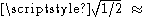0.707 of) the maximum value, or more rarely 10 dB below, or it could mean below a certain absolute value. As with any definition of the width of a function, many definitions are suitable for different purposes.

Bandwidth typically refers to baseband bandwidth in the context of, for example, sampling theorem and Nyquist sampling rate
Nyquist rate
In signal processing, the Nyquist rate, named after Harry Nyquist, is two times the bandwidth of a bandlimited signal or a bandlimited channel...

, while it refers to passband bandwidth in the context of Nyquist symbol rate
Nyquist rate
In signal processing, the Nyquist rate, named after Harry Nyquist, is two times the bandwidth of a bandlimited signal or a bandlimited channel...

or Shannon-Hartley channel capacity
Channel capacity
In electrical engineering, computer science and information theory, channel capacity is the tightest upper bound on the amount of information that can be reliably transmitted over a communications channel...

for communication systems.

## X-dB bandwidth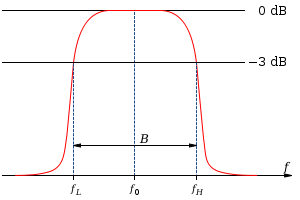In some contexts, the signal bandwidth in hertz
Hertz
The hertz is the SI unit of frequency defined as the number of cycles per second of a periodic phenomenon. One of its most common uses is the description of the sine wave, particularly those used in radio and audio applications....

refers to the frequency range in which the signal's spectral density
Spectral density
In statistical signal processing and physics, the spectral density, power spectral density , or energy spectral density , is a positive real function of a frequency variable associated with a stationary stochastic process, or a deterministic function of time, which has dimensions of power per hertz...

is nonzero or above a small threshold value. That definition is used in calculations of the lowest sampling rate that will satisfy the sampling theorem. Because this range of non-zero amplitude may be very broad or infinite, this definition is typically relaxed so that the bandwidth is defined as the range of frequencies in which the signal's spectral density is above a certain threshold relative to its maximum. Most commonly, bandwidth refers to the 3-dB bandwidth, that is, the frequency range within which the spectral density
Spectral density
In statistical signal processing and physics, the spectral density, power spectral density , or energy spectral density , is a positive real function of a frequency variable associated with a stationary stochastic process, or a deterministic function of time, which has dimensions of power per hertz...

(in W/Hz or V2/Hz) is above half its maximum value (or the spectral amplitude, in V or V/Hz, is more than 70.7% of its maximum); that is, above −3 dB
Decibel
The decibel is a logarithmic unit that indicates the ratio of a physical quantity relative to a specified or implied reference level. A ratio in decibels is ten times the logarithm to base 10 of the ratio of two power quantities...

relative to the peak.

The word bandwidth applies to signals as described above, but it could also apply to systems, for example filter
Electronic filter
Electronic filters are electronic circuits which perform signal processing functions, specifically to remove unwanted frequency components from the signal, to enhance wanted ones, or both...

s or communication channels. To say that a system has a certain bandwidth means that the system can process signals of that bandwidth, or that the system reduces the bandwidth of a white noise input to that bandwidth.

The 3 dB bandwidth of an electronic filter
Electronic filter
Electronic filters are electronic circuits which perform signal processing functions, specifically to remove unwanted frequency components from the signal, to enhance wanted ones, or both...

or communication channel is the part of the system's frequency response that lies within 3 dB of the response at its peak, which in the passband filter case is typically at or near its center frequency
Center frequency
In electrical engineering and telecommunications, the center frequency of a filter or channel is a measure of a central frequency between the upper and lower cutoff frequencies...

, and in the lowpass filter is near 0 hertz. If the maximum gain is 0 dB, the 3 dB gain is the range where the gain is more than -3dB, or the attenuation is less than + 3dB. This is also the range of frequencies where the amplitude gain is above 70.7% of the maximum amplitude gain, and above half the maximum power gain. This same "half power gain" convention is also used in spectral width
Spectral width
In telecommunications, spectral width is the wavelength interval over which the magnitude of all spectral components is equal to or greater than a specified fraction of the magnitude of the component having the maximum value....

, and more generally for extent of functions as full width at half maximum
Full width at half maximum
Full width at half maximum is an expression of the extent of a function, given by the difference between the two extreme values of the independent variable at which the dependent variable is equal to half of its maximum value....

(FWHM).

In electronic filter
Electronic filter
Electronic filters are electronic circuits which perform signal processing functions, specifically to remove unwanted frequency components from the signal, to enhance wanted ones, or both...

design, a filter specification may require that within the filter passband
Passband
A passband is the range of frequencies or wavelengths that can pass through a filter without being attenuated.A bandpass filtered signal , is known as a bandpass signal, as opposed to a baseband signal....

, the gain is nominally 0 dB +/- a small number of dB, for example within the +/- 1 dB interval. In the stopband
Stopband
A stopband is a band of frequencies, between specified limits, through which a circuit, such as a filter or telephone circuit, does not allow signals to pass, or the attenuation is above the required stopband attenuation level...

(s), the required attenuation in dB is above a certain level, for example >100 dB. In a transition band
Transition band
The transition band is a range of frequencies, that allows a transition between a passband and a stopband of a signal processing filter. The transition band is defined by a passband and a stopband cutoff frequency or corner frequency....

the gain is not specified. In this case, the filter bandwidth corresponds to the passband width, which in this example is the 1dB-bandwidth. If the filter shows amplitude ripple within the passband, the x dB point refers to the point where the gain is x dB below the nominal passband gain rather than x dB below the maximum gain.

A commonly used quantity is fractional bandwidth. This is the bandwidth of a device divided by its center frequency. E.g., a passband filter that has a bandwidth of 2 MHz with center frequency 10 MHz will have a fractional bandwidth of 2/10, or 20%.

In communication systems, in calculations of the Shannon–Hartley channel capacity
Channel capacity
In electrical engineering, computer science and information theory, channel capacity is the tightest upper bound on the amount of information that can be reliably transmitted over a communications channel...

, bandwidth refers to the 3dB-bandwidth. In calculations of the maximum symbol rate
Symbol rate
In digital communications, symbol rate is the number of symbol changes made to the transmission medium per second using a digitally modulated signal or a line code. The Symbol rate is measured in baud or symbols/second. In the case of a line code, the symbol rate is the pulse rate in pulses/second...

, the Nyquist sampling rate, and maximum bit rate according to the Hartley formula, the bandwidth refers to the frequency range within which the gain is non-zero, or the gain in dB is below a very large value.

The fact that in equivalent baseband
Baseband
In telecommunications and signal processing, baseband is an adjective that describes signals and systems whose range of frequencies is measured from close to 0 hertz to a cut-off frequency, a maximum bandwidth or highest signal frequency; it is sometimes used as a noun for a band of frequencies...

models of communication systems, the signal spectrum consists of both negative and positive frequencies, can lead to confusion about bandwidth, since they are sometimes referred to only by the positive half, and one will occasionally see expressions such as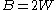, where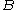is the total bandwidth (i.e. the maximum passband bandwidth of the carrier-modulated RF signal and the minimum passband bandwidth of the physical passband channel), and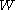is the positive bandwidth (the baseband bandwidth of the equivalent channel model). For instance, the baseband model of the signal would require a lowpass filter with cutoff frequency of at leastto stay intact, and the physical passband channel would require a passband filter of at leastto stay intact.

In signal processing and control theory
Control theory
Control theory is an interdisciplinary branch of engineering and mathematics that deals with the behavior of dynamical systems. The desired output of a system is called the reference...

the bandwidth is the frequency at which the closed-loop system gain drops 3 dB below peak.

In basic electric circuit theory, when studying band-pass and band-reject filters, the bandwidth represents the distance between the two points in the frequency domain where the signal is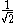of the maximum signal amplitude (half power).

## Antenna systems

In the field of antennas, two different methods of expressing relative bandwidth are used for narrowband
Narrowband
In radio, narrowband describes a channel in which the bandwidth of the message does not significantly exceed the channel's coherence bandwidth. It is a common misconception that narrowband refers to a channel which occupies only a "small" amount of space on the radio spectrum.The opposite of...

and wideband
Wideband
In communications, wideband is a relative term used to describe a wide range of frequencies in a spectrum. A system is typically described as wideband if the message bandwidth significantly exceeds the channel's coherence bandwidth....

antennas. For either, a set of criteria is established to define the extents of the bandwidth, such as input impedance
Input impedance
The input impedance of an electrical network is the equivalent impedance "seen" by a power source connected to that network. If the source provides known voltage and current, such impedance can be calculated using Ohm's Law...

, pattern
Pattern
A pattern, from the French patron, is a type of theme of recurring events or objects, sometimes referred to as elements of a set of objects.These elements repeat in a predictable manner...

, or polarization.

Percent bandwidth, usually used for narrowband
Narrowband
In radio, narrowband describes a channel in which the bandwidth of the message does not significantly exceed the channel's coherence bandwidth. It is a common misconception that narrowband refers to a channel which occupies only a "small" amount of space on the radio spectrum.The opposite of...

antennas, is used defined as. The theoretical limit to percent bandwidth is 200%, which occurs for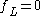.

Fractional bandwidth or Ratio bandwidth, usually used for wideband
Wideband
In communications, wideband is a relative term used to describe a wide range of frequencies in a spectrum. A system is typically described as wideband if the message bandwidth significantly exceeds the channel's coherence bandwidth....

antennas, is defined as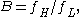and is typically presented in the form of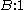. Fractional bandwidth is used for wideband antennas because of the compression of the percent bandwidth that occurs mathematically with percent bandwidths above 100%, which corresponds to a fractional bandwidth of 3:1.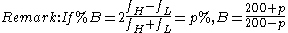.

## Photonics

In photonics
Photonics
The science of photonics includes the generation, emission, transmission, modulation, signal processing, switching, amplification, detection and sensing of light. The term photonics thereby emphasizes that photons are neither particles nor waves — they are different in that they have both particle...

, the term bandwidth occurs in a variety of meanings:
• the bandwidth of the output of some light source, e.g., an ASE source or a laser; the bandwidth of ultrashort optical pulses can be particularly large
• the width of the frequency range that can be transmitted by some element, e.g. an optical fiber
• the gain bandwidth of an optical amplifier
• the width of the range of some other phenomenon (e.g., a reflection, the phase matching of a nonlinear process, or some resonance)
• the maximum modulation frequency (or range of modulation frequencies) of an optical modulator
• the range of frequencies in which some measurement apparatus (e.g., a powermeter) can operate
• the data rate
Data rate
Data rate can refer to:* Bit rate, or data transfer rate* Data signaling rate* Data rate units-See also:* Baud rate* Channel capacity* Throughput* Bandwidth everything in this page is falsified...

(e.g., in Gbit/s) achieved in an optical communication system; see bandwidth (computing)
Bandwidth (computing)
In computer networking and computer science, bandwidth, network bandwidth, data bandwidth, or digital bandwidth is a measure of available or consumed data communication resources expressed in bits/second or multiples of it .Note that in textbooks on wireless communications, modem data transmission,...

.

A related concept is the spectral linewidth
Spectral linewidth
The spectral linewidth characterizes the width of a spectral line, such as in the electromagnetic emission spectrum of an atom, or the frequency spectrum of an acoustic or electronic system...

of the radiation emitted by excited atoms.

• Bandwidth (Wiktionary entry)
• Bandwidth efficiency
• Bandwidth extension
Bandwidth extension
Bandwidth extension of signal is defined as the deliberate process of expanding the frequency range of a signal in which it contains an appreciable and useful content, and/or the frequency range in which its effects are such...

The term broadband refers to a telecommunications signal or device of greater bandwidth, in some sense, than another standard or usual signal or device . Different criteria for "broad" have been applied in different contexts and at different times...

• Frequency
Frequency
Frequency is the number of occurrences of a repeating event per unit time. It is also referred to as temporal frequency.The period is the duration of one cycle in a repeating event, so the period is the reciprocal of the frequency...

• Narrowband
Narrowband
In radio, narrowband describes a channel in which the bandwidth of the message does not significantly exceed the channel's coherence bandwidth. It is a common misconception that narrowband refers to a channel which occupies only a "small" amount of space on the radio spectrum.The opposite of...

• Modulation
Modulation
In electronics and telecommunications, modulation is the process of varying one or more properties of a high-frequency periodic waveform, called the carrier signal, with a modulating signal which typically contains information to be transmitted...

• Shannon–Hartley theorem
Shannon–Hartley theorem
In information theory, the Shannon–Hartley theorem tells the maximum rate at which information can be transmitted over a communications channel of a specified bandwidth in the presence of noise. It is an application of the noisy channel coding theorem to the archetypal case of a continuous-time...

gives the channel capacity
Channel capacity
In electrical engineering, computer science and information theory, channel capacity is the tightest upper bound on the amount of information that can be reliably transmitted over a communications channel...

• Wideband
Wideband
In communications, wideband is a relative term used to describe a wide range of frequencies in a spectrum. A system is typically described as wideband if the message bandwidth significantly exceeds the channel's coherence bandwidth....